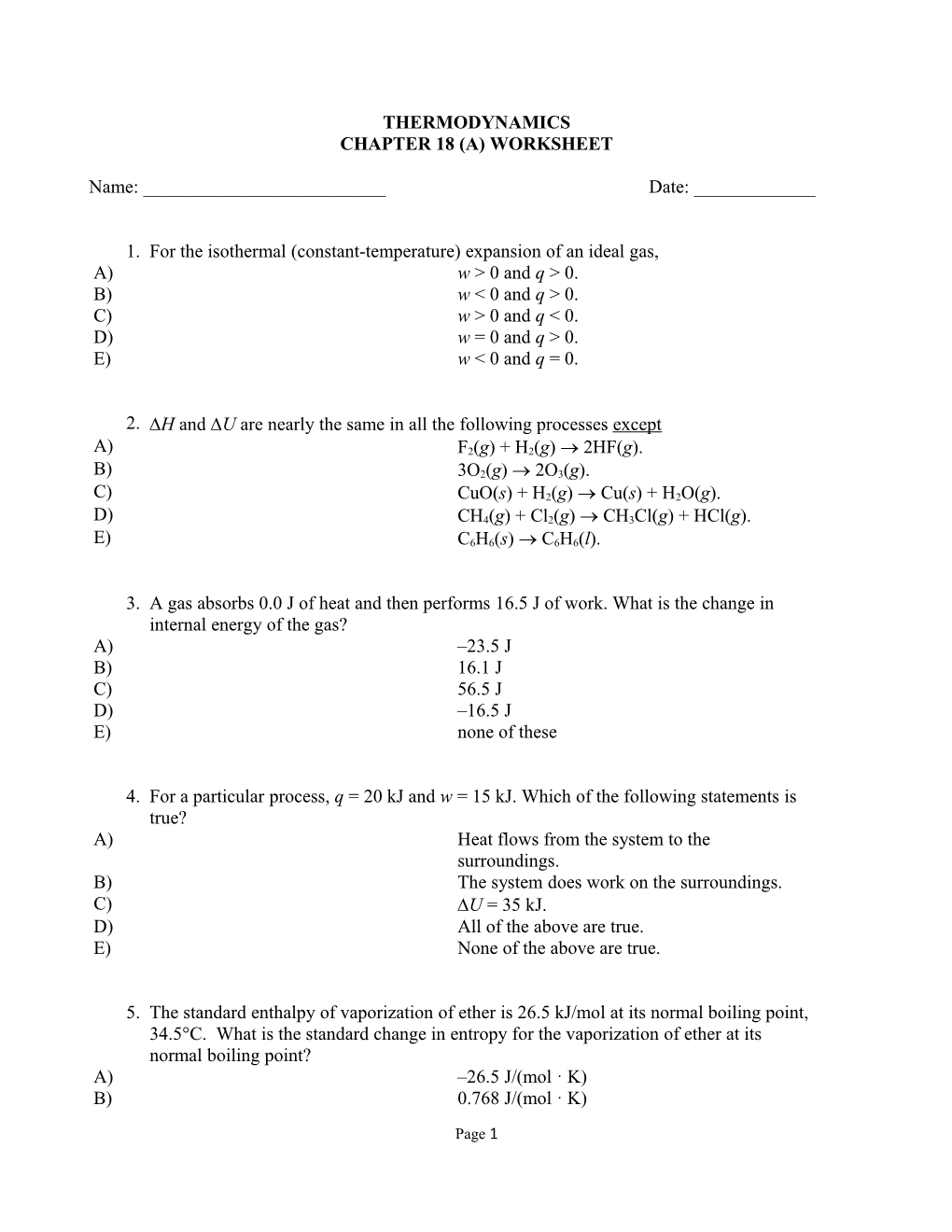# Chapter 18 (A) WorksheetTHERMODYNAMICS

CHAPTER 18 (A) WORKSHEET

Name: ______Date: ______

1. / For the isothermal (constant-temperature) expansion of an ideal gas,
A) / w > 0 and q > 0.
B) / w < 0 and q > 0.
C) / w > 0 and q < 0.
D) / w = 0 and q > 0.
E) / w < 0 and q = 0.
2. / H and U are nearly the same in all the following processes except
A) / F2(g) + H2(g)  2HF(g).
B) / 3O2(g)  2O3(g).
C) / CuO(s) + H2(g)  Cu(s) + H2O(g).
D) / CH4(g) + Cl2(g)  CH3Cl(g) + HCl(g).
E) / C6H6(s)  C6H6(l).
3. / A gas absorbs 0.0 J of heat and then performs 16.5 J of work. What is the change in internal energy of the gas?
A) / –23.5 J
B) / 16.1 J
C) / 56.5 J
D) / –16.5 J
E) / none of these
4. / For a particular process, q = 20 kJ and w = 15 kJ. Which of the following statements is true?
A) / Heat flows from the system to the surroundings.
B) / The system does work on the surroundings.
C) / U = 35 kJ.
D) / All of the above are true.
E) / None of the above are true.
5. / The standard enthalpy of vaporization of ether is 26.5 kJ/mol at its normal boiling point, 34.5°C. What is the standard change in entropy for the vaporization of ether at its normal boiling point?
A) / –26.5 J/(mol · K)
B) / 0.768 J/(mol · K)
C) / 768 J/(mol · K)
D) / 86.1 J/(mol · K)
E) / 0.0861 J/(mol · K)
6. / The standard enthalpy of fusion of iodobenzene is 9.75 kJ/mol at its melting point, 241.8 K. What is the standard change in entropy for the melting of iodobenzene at its melting point?
A) / –9.75 J/(mol · K)
B) / –311 J/(mol · K)
C) / 0.0403 J/(mol · K)
D) / 40.3 J/(mol · K)
E) / 0.0189 J/(mol · K)
7. / For which of the following processes would S° be expected to be most positive?
A) / O2(g) + 2H2(g)  2H2O(g)
B) / H2O(l)  H2O(s)
C) / NH3(g) + HCl(g)  NH4Cl(g)
D) / 2NH4NO3(s)  2N2(g) + O2(g) + 4H2O(g)
E) / N2O4(g)  2NO2(g)
8. / What is S° at 298 K for the following reaction?
2O3(g)  3O2(g)
Substance / S° (J/(mol · K)) at 298 K
O2(g) / 205.0
O3(g) / 238.8
A) / +137.4 J/K
B) / –137.4 J/K
C) / +33.8 J/K
D) / –33.8 J/K
E) / +443.8 J/K
9. / What is the change in entropy when 7.67 mL of liquid benzene (C6H6, d = 0.879 g/mL) is combusted in the presence of 12.7 L of oxygen gas, measured at 298 K and 1 atm pressure? (R = 0.0821 L · atm/(K · mol))
2C6H6(l) + 15O2(g) → 12CO2(g) + 6H2O(l); ∆S° = –437.7 J/K at 298 K
A) / –265 J/K
B) / –227 J/K
C) / –37.8 J/K
D) / –34.0 J/K
E) / –15.1 J/K
10. / Consider the following hypothetical reaction at 310 K. Standard free energies of formation are given in parentheses.
B CG° = –28.3 kJ/mol
(?)(176.4 kJ/mol)
Calculate the standard free energy of formation of compound B.
A) / 204.7 kJ/mol
B) / –204.7 kJ/mol
C) / 148.1 kJ/mol
D) / –148.1 kJ/mol
E) / none of these
11. / Consider the following hypothetical reaction (at 310.0 K). Standard free energies, in kJ/mol, are given in parentheses.
AB+CG° = ?
(–32.2) (207.8) (–237.0)
What is the value of the equilibrium constant for the reaction at 310.0 K?
A) / 0.31
B) / 1.0
C) / 8.3 × 104
D) / 273
E) / 0.42
12. / What is G° at 500.0 K for the following reaction?
Ba(s) + H2O(g)  BaO(s) + H2(g)
Substance / H°f (kJ/mol) at 298 K / S° (J/(mol · K)) at 298 K
Ba(s) / 0 / 62.8
H2O(g) / –241.8 / 188.7
BaO(s) / –592.0 / 70.4
H2(g) / 0 / 130.6
A) / –324.9 kJ
B) / –335.2 kJ
C) / 324.9 kJ
D) / 335.2 kJ
E) / –375.4 kJ
13. / The reaction CaO(s) + SO3(g)  CaSO4(s) is nonspontaneous at 2200 K, whereas it is spontaneous at room temperature. Which of the following statements is false?
A) / G is negative at room temperature.
B) / Both H and S are negative for the reaction.
C) / G becomes zero at a temperature between 300 and 2200 K.
D) / The change in enthalpy is the main driving force of the reaction.
E) / The change in entropy is the main driving force of the reaction.
14. / The reaction C(s) + CO2(g)  2CO(g) is spontaneous only at temperatures in excess of 1100 K. We can conclude that
A) / H° is positive and S° is negative.
B) / H° is negative and S° is negative.
C) / H° is positive and S° is positive.
D) / H° is negative and S° is positive.
E) / G° is negative for all temperatures.

1. / B
2. / B
3. / D
4. / C
5. / D
6. / D
7. / D
8. / A
9. / E
10. / A
11. / A
12. / A
13. / E
14. / C

Page 1